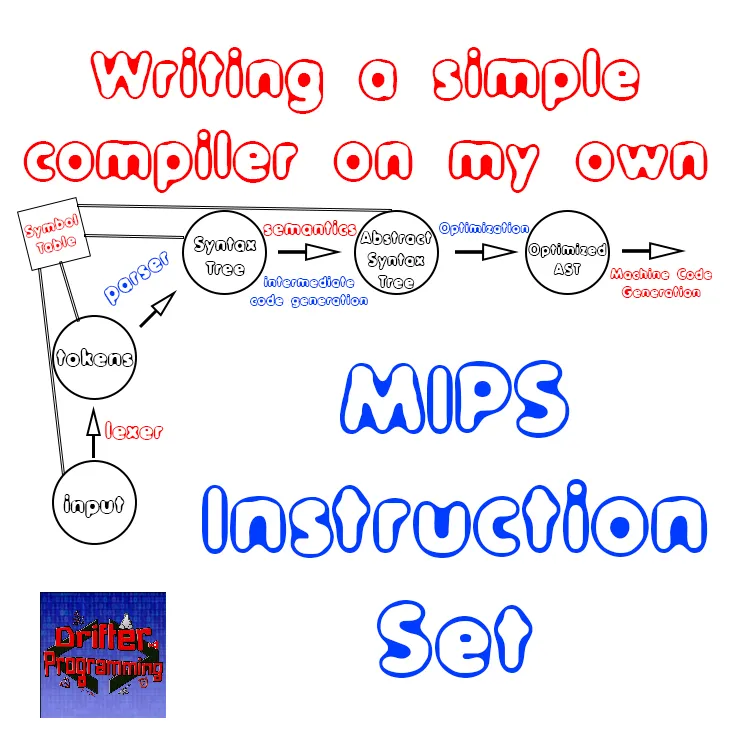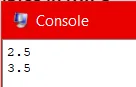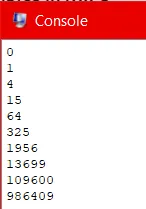# Writing a simple Compiler on my own - Simple Examples in MIPS Assembly[Custom Thumbnail]

All the Code of the series can be found at the Github repository:
https://github.com/drifter1/compiler

# Introduction

Hello it's a me again @drifter1! Today we continue with my Compiler Series, a series where we implement a complete compiler for a simple C-like language by using the C-tools Flex and Bison. In this article we will write the simple examples that we used through-out the series in MIPS Assembly Code. To understand this article, I highly suggest you to go read my previous article about the MIPS Instruction Set...

## Requirements:

Actually you need to read and understand all the topics that I covered in the series as a whole, as these articles will give you access to knowledge about:
• What Compiler Design is (mainly the steps)
• For which exact Language the Compiler is build for (Tokens and Grammar)
• How to use Flex and Bison
• How to implement a lexer and parser for the language using those tools
• What the Symbol Table is and how we implement it
• How we combine Flex and Bison together
• How we can pass information from the Lexer to the Parser
• How we define operator priorities, precedencies and associativity
• What Semantic Analysis is (Attributes, SDT etc.)
• How we do the so called "Scope Resolution"
• How we declare types and check the type inter-compatibility for different cases that include function parameters and assignments
• How we check function calls later on using a "Revisit queue", as function declarations mostly happen after functions get used (in our Language)
• Intermediate Code Representations, including AST's
• How we implement an AST (structure and management)
• Action Rules for AST nodes and more
• The target system's architecture and its assembly language (MIPS)

## Difficulty:

Talking about the series in general this series can be rated:
Today's topic(s) can be rated:
• Medium

# Actual Tutorial Content

## Example 1

The first example code that we saw during the series had no functions and did only one simple calculation using two variables. More specifically, we just define three variables: one integer i and two doubles val and res, and initialize the double val to 2.5 and set the value of the other double res to be equal to the value of val plus 1 (res = val + 1). The integer i is of no use, but still worth mentioning as you can see how we declare such a variable...

Either way, the code that I'm talking about is:
``````// declarations
int i;
double val, res;
// statements
val = 2.5;
res = val + 1;
return;``````

So, how do we turn this Simple-C code into MIPS Assembly?

### Data Declarations

An integer takes up 4 bytes of space, which are called a word and so we can use the keyword ".word" to define the integer. For the first one we of course also have to give an initialization value, let's say zero (0). On the other hand, an double takes up 8 bytes of space, that we will have to input manually using the keyword ".space". For testing purposes we will also define a new line message: "\n", so that we can print out the values of the two doubles in two different lines of the console.

Therefore, the ".data" section is:
``````.data
i:     .word   0
val:     .space  8   # \$f0
res:     .space  8   # \$f2
newline: .asciiz "\n"``````
In the comments of this code you can also see that I "map" val to the register \$f0 and res to the register \$f2, as the arithmetic operation of addition has to be applied on the FP registers and cannot be applied on the memory.

### Instructions

So, now for the main instructions-section. First, we should initialize the variable val to 2.5, by setting the floating point register \$f0 and the space that we declared previously to that value. This can be done quite simply by loading the immediate value 2.5 to the register \$f0 and then storing the register to the memory space that we allocated for this variables. In other word we write:
``````li.d \$f0, 2.5
s.d \$f0, val``````

Next up, is the addition of 1 to the value of the register val, that will give us the value of res (result). For that we will have to load the immediate value 1.0 into another register, so that we can use that value in the add instruction. So, loading the value 1.0 to register \$f4 and adding that value to the value of \$f0 and storing this value to \$f2, we are basically done. In the end, \$f2 will contain the result of val + 1. After that, we can also store that result to memory. So, we have:
``````li.d \$f4, 1.0
s.d \$f2, res``````

After that we can do 3 system calls, to print out the 2 doubles and a new line character in-between of each of those prints. In code, this looks like this:
``````li \$v0, 3
mov.d \$f12, \$f0
syscall
li \$v0, 4
la \$a0, newline
syscall
li \$v0, 3
mov.d \$f12, \$f2
syscall``````

Finally, we also have to terminate the program writing:
``````li \$v0, 10
syscall``````

### Console Output

The final MIPS code for this program is:
``````.data
i:     .word   0
val:     .space  8   # \$f0
res:     .space  8   # \$f2
newline: .asciiz "\n"
.text
main:
# val = 2.5
li.d \$f0, 2.5
s.d \$f0, val
# res = val + 1
li.d \$f4, 1.0
s.d \$f2, res
# print values for testing
li \$v0, 3
mov.d \$f12, \$f0
syscall
li \$v0, 4
la \$a0, newline
syscall
li \$v0, 3
mov.d \$f12, \$f2
syscall
# terminate program
li \$v0, 10
syscall``````

Running it in QtSpim we get:2.5 is the value of val, whilst 3.5 is the value of res.

## Example 2

After this warm-up, let's now get into a more interesting example, which is example2.c. The code of this example is:
``````// declarations
int i;
double val = 2.5, res;
// statements
for(i = 0; i < 10; i++){
res[i] = operation(val, i);
val = res[i];
print(res[i]);
print('\n');
}
return;
// functions
double operation (double value, int i){
// declarations
double res;
// statements
res = value*i + i;
return res;
}``````

Let's again start by declaring the variables...

### Data declaration

Here we have one integer, one double and one double array of 10 entries. Taking a look at the print() functions that occur through-out the code, we can also see that a "\n" message is needed and so we will use the same that we define previously. So, similar to the previous example, we will define the integer i as a ".word" with initial value 0, the double val as a ".space" of byte-size 8 and lastly the array will be a ".space" of size 80, so that it can store 10 doubles (10x8 = 80).

The ".data" section's code thus is:
``````.data
i:     .word   0   # \$f4 as FP
val:     .space  8   # \$f2
res:     .space  80  # array with 10 doubles
newline: .asciiz "\n"``````

You can again see how I "map" the various variables to registers. As we are talking about floating point operations, the integer i will have to be converted to a double, and so we map it to the FP register \$f4. Similar to before we also define a register for val, supposing that the variable will be stored inside of \$f2.

### Instructions

Similar to before, we have to initialize the variable val to 2.5, by setting the register \$f2 to 2.5 and storing that value to the memory space that we allocated for that purpose. So, the first part of the ".text" section is:
``````.text
main:
# val = 2.5
li.d \$f2, 2.5
s.d \$f2, val``````

After that we get to meet our friend the for-loop (!).As we have to store values back to the array, but also have to use the loop counter by itself, we basically have to define two loop counters that will increment simultaneously. The register \$t0 will be the "actual" loop counter that will go from 0 to 9 in steps of 1, whilst \$t1 will be an address counter that will go from 0 to 72, incrementing in steps of 8. The exit condition of value 10 will be stored in the register \$t2.

So, knowing that the value of \$t0 needs to be less than the value 10, we will use the reverse-logic to branch out of the loop when the value of the counter becomes 10 or more (greater equal). Thus, we will use the branch if greater equal (bge) branch instruction. At the end of the for loop's body we will jump back to the start using a simple j-Jump. So, the basic structure of our for-loop is:
``````# for loop
li \$t0, 0   # i = 0
li \$t1, 0   # address counter
li \$t2, 10  # exit condition
for: bge \$t0, \$t2, end_for  # i < 10
....
other instructions
...
# i++
j for
end_for:``````

The operands/parameters of the "operation"-function are stored in \$f2 and \$t0, and so we can easily write the function. In a separate label below the main function we will have to convert the int to a double, storing the value of i in a FP register, let's say \$f4. After that we can easily apply the value*i = i operation and return the result in another FP register, let's say \$f0. In the end, we have to return back using jr \$ra, as \$ra stores the address of the instruction after the function call, that is done using jal. Either way, the operation function looks like this:
``````operation:
# convert int to double
mtc1.d \$t0, \$f4
cvt.d.w \$f4, \$f4
# \$f4 contains i
# res = value*i + i
mul.d \$f0, \$f2, \$f4
# return value in \$f0
jr \$ra``````

Don't be to scared about that first part! We simply, move a value from the general-purpose registers of the MIPS CPU to the Co-processor that does floating point operations. So, the value of \$t0 gets stored into \$f4. The second instruction, basically converts the integer value into a double value. The rest is easier to understand, I think.

So, getting back to the for-loop's body, we call the function that we defined seconds ago using "jal operation", and then simply store the result to the res array, using the store instruction "s.d". After that we also have to "move" that value into the val register \$f2, by also storing this value into memory (lots of optimizations could be made here, as only storing into the array is necessary). The code for these three things is:
``````# temp = operation(val, i)
# val in \$f2
# i in \$t0
jal operation
# res[i] = temp
s.d \$f0, res(\$t1)
# val = res[i]
mov.d \$f2, \$f0
s.d \$f2, val(\$0)
``````

After that we only have to print out the value that we got in that step, using:
``````# print(res[i])
li \$v0, 3
mov.d \$f12, \$f0
syscall
# print("\n")
li \$v0, 4
la \$a0, newline
syscall``````

### Console Output

The complete code looks like this:
``````.data
i:     .word   0   # \$f4 as FP
val:     .space  8   # \$f2
res:     .space  80  # array with 10 doubles
newline: .asciiz "\n"
.text
main:
# val = 2.5
li.d \$f2, 2.5
s.d \$f2, val
# for loop
li \$t0, 0   # i = 0
li \$t1, 0   # address counter
li \$t2, 10  # exit condition
for: bge \$t0, \$t2, end_for  # i < 10
# temp = operation(val, i)
# val in \$f2
# i in \$t0
jal operation
# res[i] = temp
s.d \$f0, res(\$t1)
# val = res[i]
mov.d \$f2, \$f0
s.d \$f2, val(\$0)
# print(res[i])
li \$v0, 3
mov.d \$f12, \$f0
syscall
# print("\n")
li \$v0, 4
la \$a0, newline
syscall
# i++
j for
end_for:
# terminate program
li \$v0, 10
syscall
operation:
# convert int to double
mtc1.d \$t0, \$f4
cvt.d.w \$f4, \$f4
# \$f4 contains i
# res = value*i + i
mul.d \$f0, \$f2, \$f4
# return value in \$f0
jr \$ra``````

Running the code in the console we get:As you can see the initial value of val is useless, as it gets multiplied by 0 and gets the final value 0. The result at each step is equal to the previous result multiplied by the current step's loop counter, that gets also added to the loop counter's value.

## RESOURCES

### References:

No references, just using code that I implemented in my previous articles.

### Images:

All of the images are custom-made!

## Previous parts of the series

### Syntax Analysis

• Syntax Analysis Theory
• Bison basics
• Creating a grammar for our Language
• Combine Flex and Bison
• Passing information from Lexer to Parser
• Finishing Off The Grammar/Parser (part 1)
• Finishing Off The Grammar/Parser (part 2)
• ### Semantic Analysis (1)

• Semantic Analysis Theory
• Semantics Examples
• Scope Resolution using the Symbol Table
• Type Declaration and Checking
• Function Semantics (part 1)
• Function Semantics (part 2)
• ### Intermediate Code Generation (AST)

• Abstract Syntax Tree Principle
• Abstract Syntax Tree Structure
• Abstract Syntax Tree Management
• Action Rules for Declarations and Initializations
• Action Rules for Expressions
• Action Rules for Assignments and Simple Statements
• Action Rules for If-Else Statements
• Action Rules for Loop Statements and some Fixes
• Action Rules for Function Declarations (part 1)
• Action Rules for Function Declarations (part 2)
• Action Rules for Function Calls
• ### Semantic Analysis (2)

• Datatype attribute for Expressions
• Type Checking for Assignments
• Revisit Queue and Parameter Checking (part 1)
• Revisit Queue and Parameter Checking (part 2)
• Revisit Queue and Parameter Checking (part 3)
• Revisit Queue and Parameter Checking (part 4)
• Revisit Queue and Assignment Checking (part 1)
• Revisit Queue and Assignment Checking (part 2)
• Revisit Queue and Assignment Checking (part 3)

## Final words | Next up on the project

And this is actually it for today's post! I hope that I explained everything as much as I needed to. Next time, we will write the "full_example.c" file in MIPS Assembly!

Next up on this series, in general, are:
• Machine Code generation (MIPS Assembly)
• Various Optimizations in the Compiler's Code

Which are all topics for which we will need more than one article to complete them. Also, note that we might also get into AST Code Optimizations later on, or that we could even extend the Language by adding complex datatypes (structs and unions), more rules etc.
So, see ya next time!

## GitHub Account:

https://github.com/drifter1Keep on drifting! ;)

H2
H3
H4
3 columns
2 columns
1 column